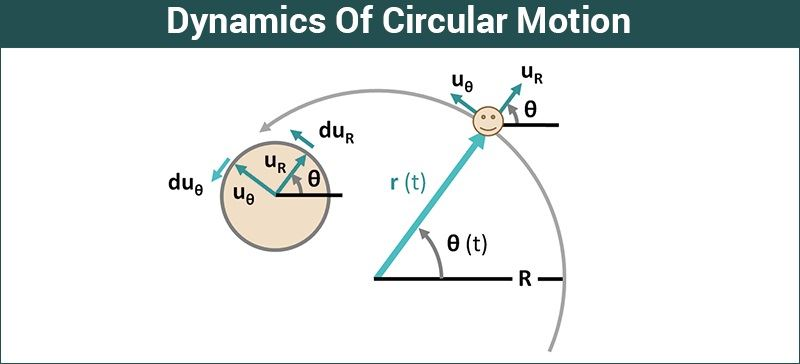# Dynamics Of Circular Motion

We know that circular motion can be both uniform and non-uniform. If the tangential component of acceleration is absent, it will be uniform circular motion, and if the tangential component of acceleration is present, it will be non-uniform circular motion. In case of non-uniform circular motion, the net acceleration of the particle is the resultant of radial acceleration and tangential acceleration.Now, suppose you are in an inertial frame of reference and you are observing a particle in a circular motion. The net force on the particle must be non-zero according to the second law of motion since the particle has some acceleration. Let’s take the case of uniform circular motion. The speed of the particle is constant, and the acceleration of the particle towards the center is

$$\begin{array}{l}\frac{v^{2}}{r}\end{array}$$
.

Let the mass of the particle be m. From the second law of Newton, we can say that

$$\begin{array}{l}F\end{array}$$
=
$$\begin{array}{l}ma\end{array}$$

$$\begin{array}{l} F \end{array}$$
=
$$\begin{array}{l} m \frac{v^{2}}{r}\end{array}$$

This force is directed towards the center and is therefore known as centripetal force. This centripetal force is required to keep the object in a uniform circular motion. This is just the name given to this type of force and this centripetal force can arise from tension, friction, etc.

When a vehicle turns on roads, they travel along a circular arc. The only forces acting on the vehicle are:

1) Weight Mg
2) Normal reaction force N
3) Friction

If the road is horizontal, the first two forces must be in a vertical direction. Therefore, the only force that can provide the radial acceleration for turning is friction. In this case, the force of friction acts as the centripetal force.

$$\begin{array}{l}f_s\end{array}$$
=
$$\begin{array}{l}M \frac{v^{2}}{r}\end{array}$$

Since there is a limit to the maximum value of friction, for a safe turn,

$$\begin{array}{l}m \frac{v^{2}}{r} \leq µ_s N\end{array}$$

where,

$$\begin{array}{l}µ_s\end{array}$$
= coefficient of static friction

### Recommended Video